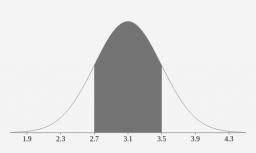# Distribution 6283

The life of the bulbs has a normal distribution with a mean value of 2000 hours and a standard deviation of 200 hours. What is the probability that the light bulb will last for at least 2100 hours?

p =  0.3085

### Step-by-step explanation:Did you find an error or inaccuracy? Feel free to write us. Thank you!

Tips for related online calculators
Looking for a statistical calculator?
Looking for a standard deviation calculator?
Would you like to compute the count of combinations?

#### Grade of the word problem:

We encourage you to watch this tutorial video on this math problem: